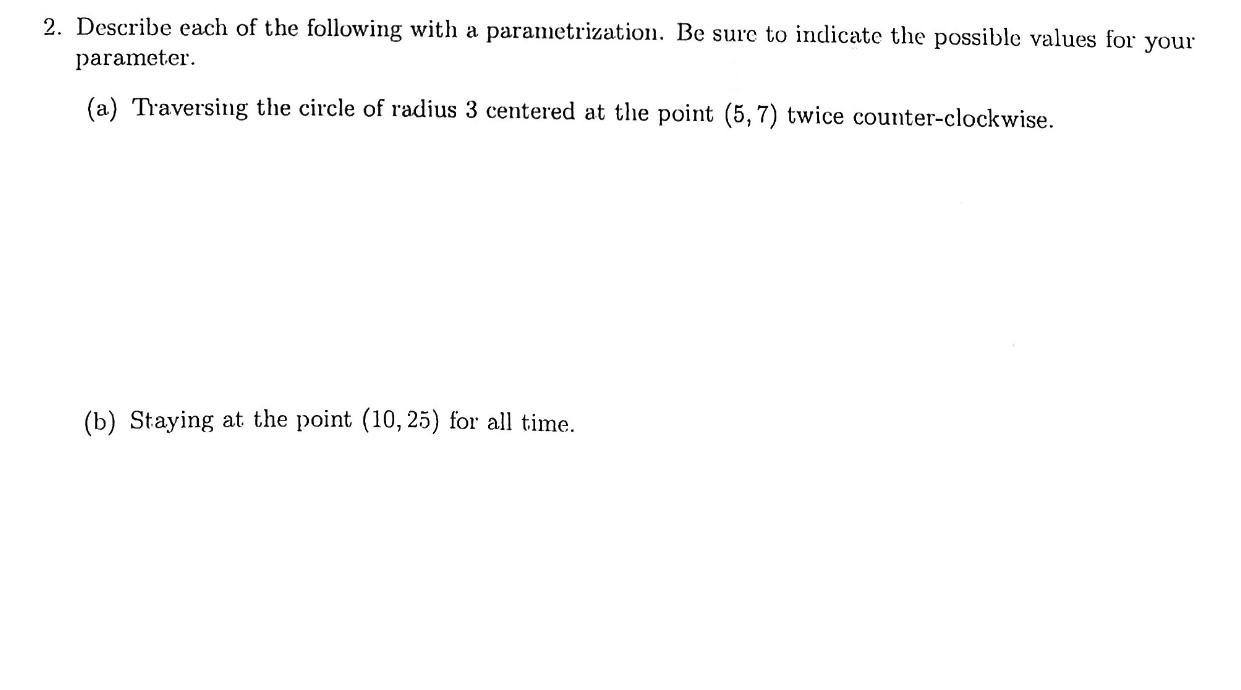# 2. Describe each of the following with a parametrization. Be sure to indicate the possible values forparameteryour(a) Traversing the circle of radius 3 centered at the point (5,7) twice counter-clockwise(b) Staying at the point (10,25) for all time

Question
114 viewshelp_outlineImage Transcriptionclose2. Describe each of the following with a parametrization. Be sure to indicate the possible values for parameter your (a) Traversing the circle of radius 3 centered at the point (5,7) twice counter-clockwise (b) Staying at the point (10,25) for all time fullscreen
check_circle

Step 1

It is given that the circle having center (5,7) has the radius 3 units.

Step 2

To find:

• The parametric equation of the given circle when centre is (5,7) and radius 3 units is rotated two times counter-clockwise.
• The parametric equation of the given circle when the centre is (10,25) and radius is 3 units.
Step 3

Since, the centre of the circle is (5,7), therefore after two times rotation it will subtend an angle of 720 degr...

### Want to see the full answer?

See Solution

#### Want to see this answer and more?

Solutions are written by subject experts who are available 24/7. Questions are typically answered within 1 hour.*

See Solution
*Response times may vary by subject and question.
Tagged in

### Circles